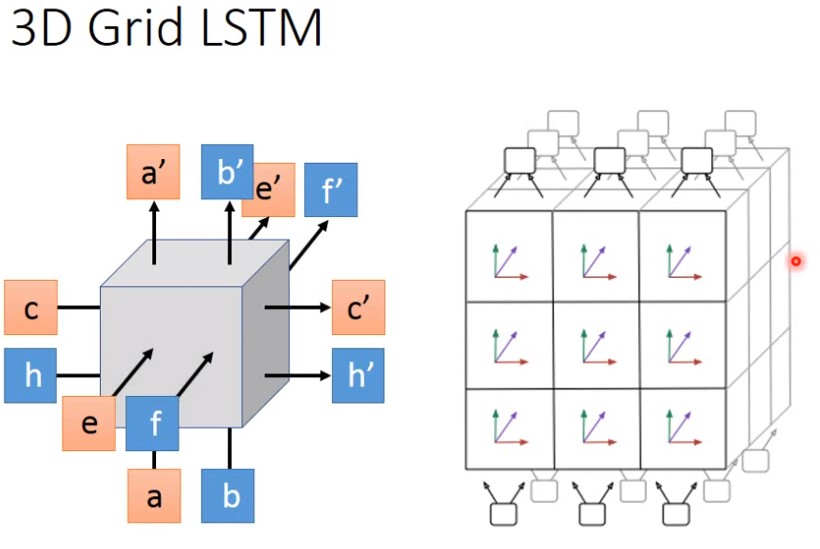李宏毅深度学习-六-HighwayNetwork和LSTM

1 前馈网络和循环神经网络(RNN)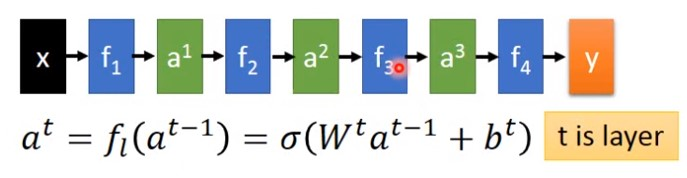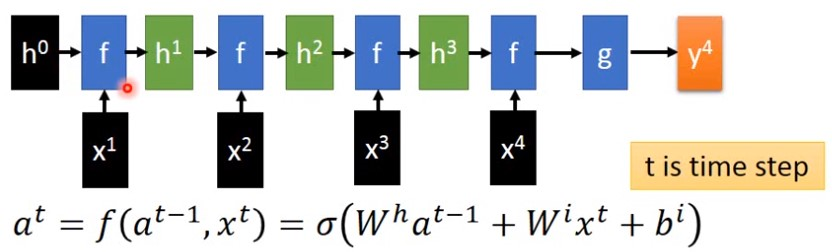1. 循环神经网络每个网络层都有输入，而前馈网络只有一个输入
2. 循环神经网络的每一层的激活函数是同一个，而前馈网络的每一层的激活函数都不同。

2 如何把GRU改成Highway Network

GRU网络示意图如下：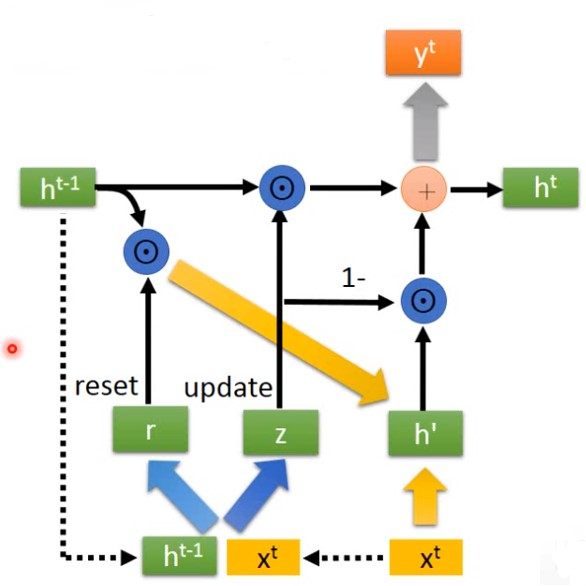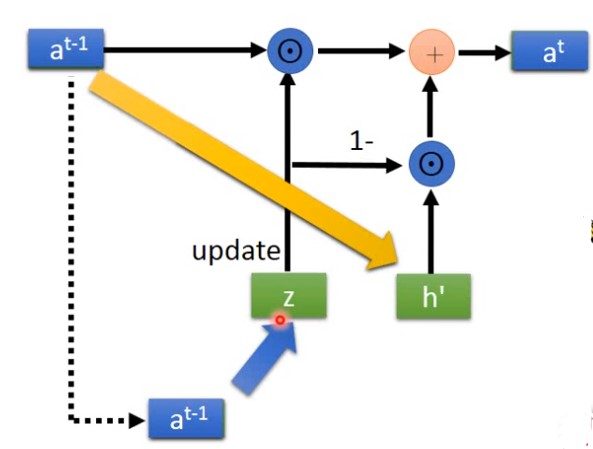1. 拿掉每个时间步的输入$x^t$,
2. 拿掉$y^t$,RNN每个时间步都会输出一个$y^t$,Highway network只有一个最后的输出$y^t$.
3. 把 $h^{t-1}$ 改成 $a^{t-1}$。其中$a^{t-1}$ 是第$t$层的输出
4. 拿掉reset gate。它的作用是让GRU忘记之前发生过的事情，但是Highway不应该忘记，它只有一个开始的。

3 Highway Network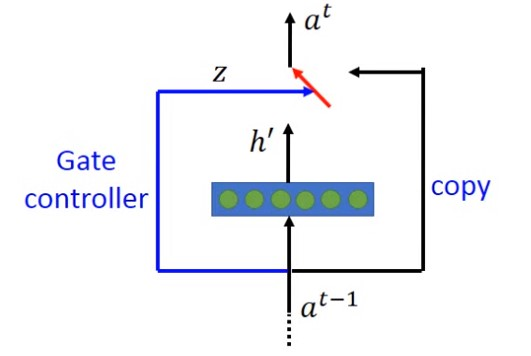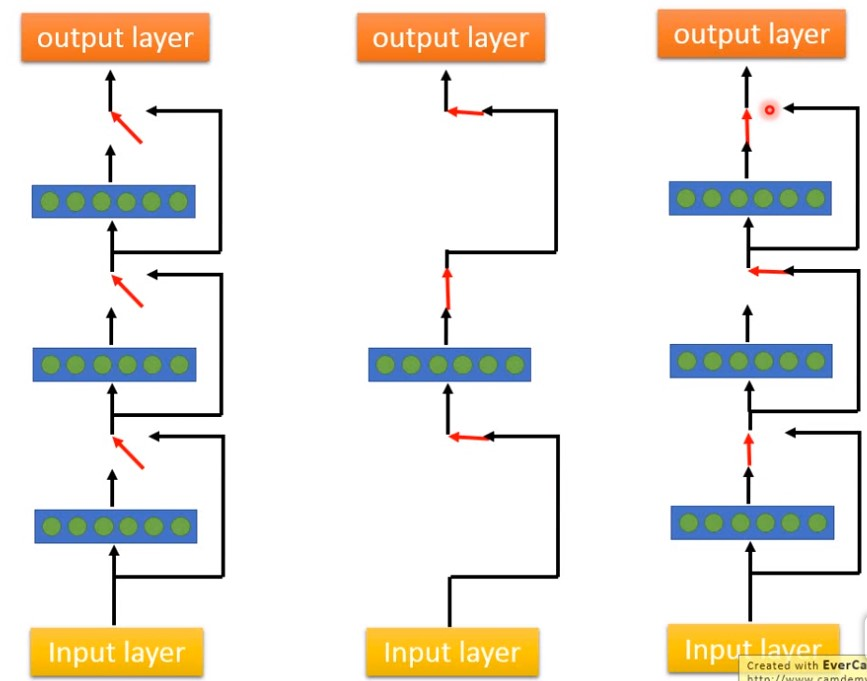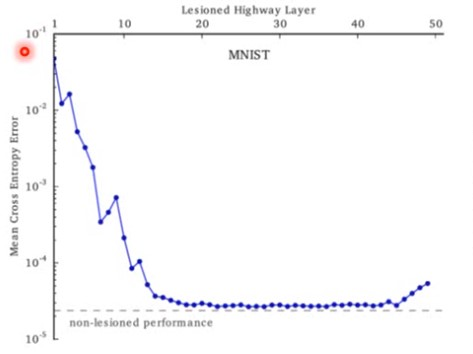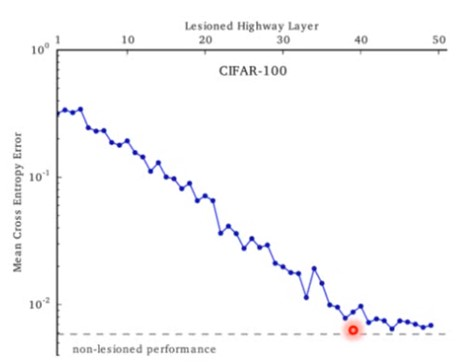4 Grid LSTM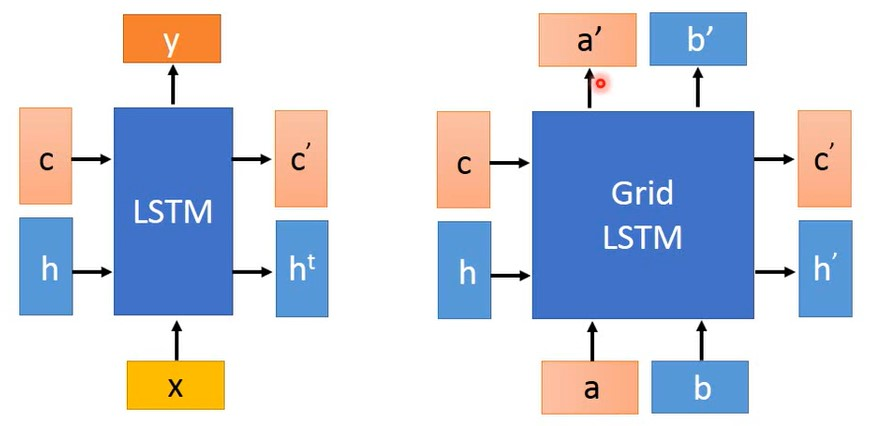Grid LSTM时间方向上与传统的LSTM一致，多出了一个深度方向的输入输出.输入是$a$,$b$输出是$a’$,$b’$

4.1 Grid LSTM如何连接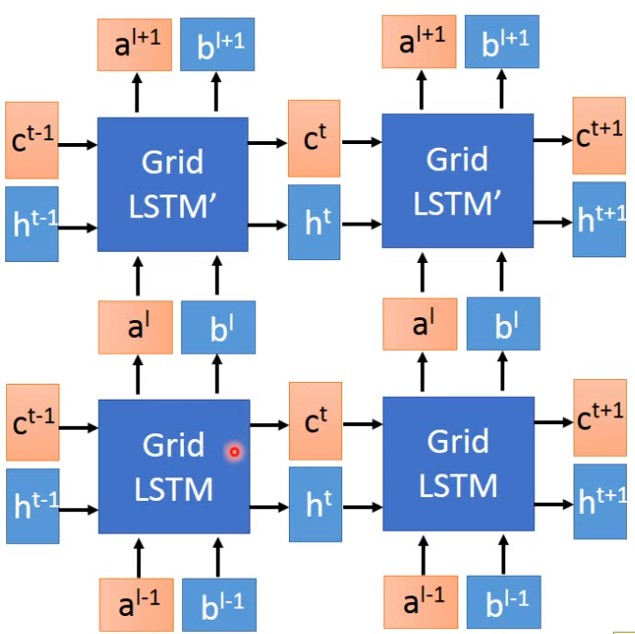4.2 Grid LSTM内部结构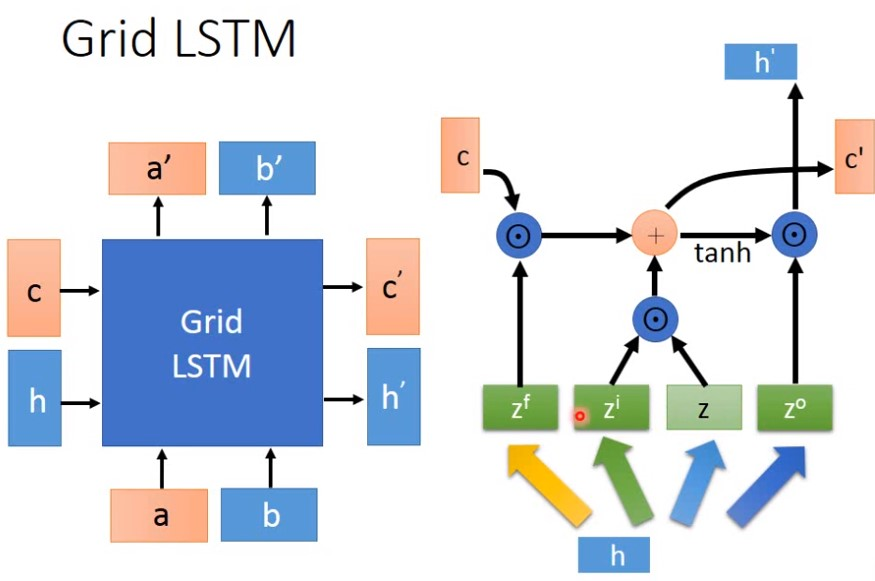• $h$ 是输入
• $z^f$ 是 遗忘门
• $z^i$ 是输入门
• $z$ 是 输入信息
• $z^o$ 是输出门
• $c$ 是记忆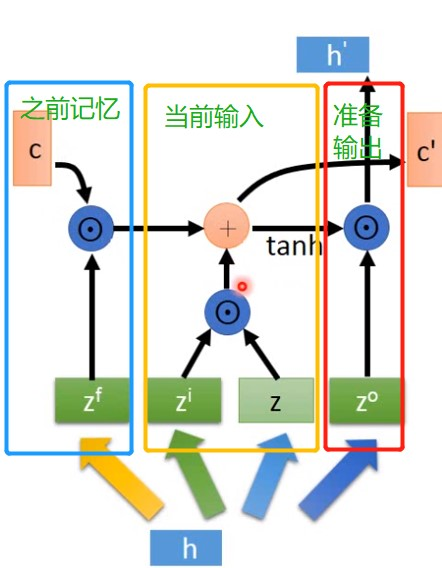4.3 Grid LSTM的输入输出

Grid LSTM 有两套记忆以及两套隐藏层输出 ，如何结合并表现出来？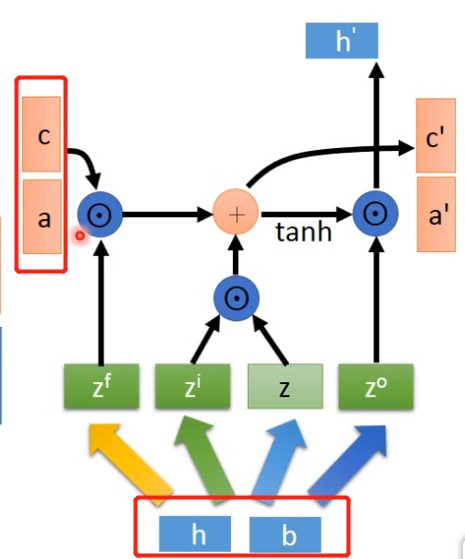4.4 3D Grid LSTM